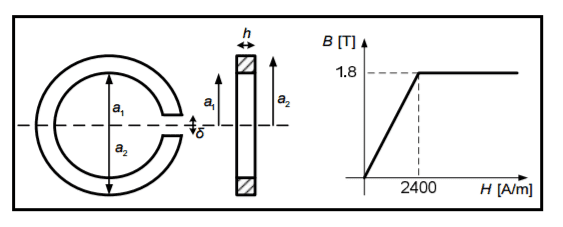# Energy of Toroidal Solenoid

## Homework Statement

[/B]
N=600 (number of loops)
a1=200mm
a2=240mm
h=40mm
δ=1,5mm or l0
S0=1,1*S (Cross sectional area)
B0=1,2T

I need to find energy of toroid and currect going through it.
Solution: W=4,08J, I=6,44A2. The attempt at a solution

So far I managed to calculate S and S0:
S=(a2-a1)*h
S=0,0016m2
S0=1,1*S
S0=0,00176m2

I tried to find energy using this formula but it's not correct:
W0=B02*S0*l0/2*μ0
W0=1,51J

W=B*H*S*(l-l0)/2 (B and H are from the graph)
W=4,76J

Formula for current is I=NH/l but since nothing is correct I didn't bother to try and calculate it.

Your formula I=NH/l is wrong however so not using it was ironically a good move!